Science, Maths & Technology

### Become an OU student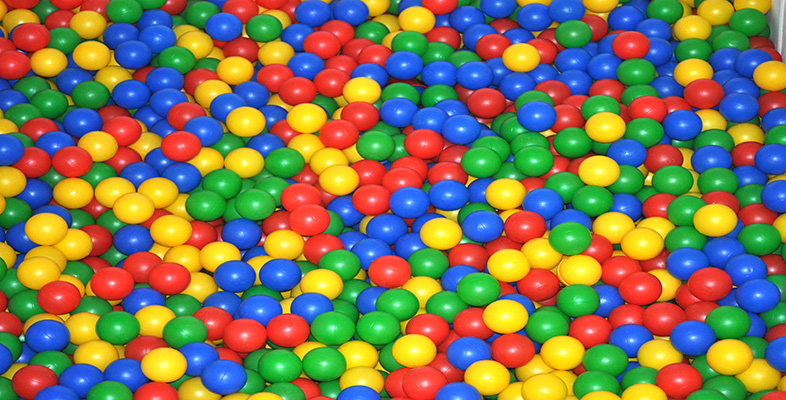Introduction to polymers

Start this free course now. Just create an account and sign in. Enrol and complete the course for a free statement of participation or digital badge if available.

# 4.5.1 The copolymer equation

It can be shown that the rate of change of monomer concentration in any copolymerization is given by the equation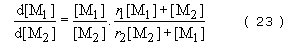where [M1] and [M2] are the concentrations of monomers 1 and 2 at any instant and r1 and r2., are reactivity ratios. The reactivity ratios represent the rate at which one type of growing chain end adds on to a monomer of the same structure relative to the rate at which it adds on to the alternative monomer. The copolymer equation can be used to predict chain structure in the three different ways, already mentioned.

The formation of regular alternating copolymers of the type ABAB… is favoured when each growing radical prefers to add to monomer of the opposite type. In this case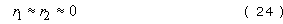and Equation (23) therefore becomes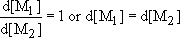In other words both monomers will disappear from the reaction vessel at the same rate.

An ideal copolymer will tend to form when each type of chain end shows an equal preference for adding on to either monomer. In this case,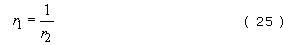and the copolymer equation becomes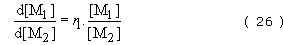Hence composition depends on the relative amounts of monomer present at any time and the relative reactivities of the two monomers.

Finally, block copolymers are formed when the growing chain end has a marked preference for adding on to the same kind of monomer. In this case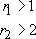As can be seen from Table 8, this is rarely achieved in free radical copolymerization. However, it is possible to form block structures in anionic polymerization simply by feeding different monomers to the living polymer. Step growth copolymerizations produce ideal (random) copolymers since in this special case r 1 = r 2 = 1.

Monomer 1Monomer 2 r1 r2